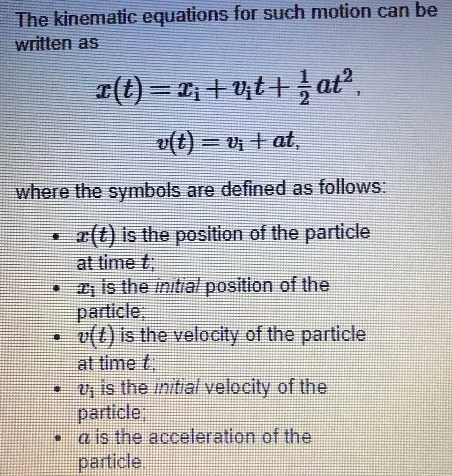# Problem: 1. The quantity represented by x is a function that changes over time (i.e.,is not constant) true or false?2. The quantity represented by xi initial position is a function of time (i.e.,is not constant) true or false?3. The quantity represented by vi initial velocity is a function of time (i.e.,not a constant) true or false?4. The quantity represented by v(t) is a function of time (i.e.,not a constant) true or false?5. A particle moves with the constant acceleration a. The expression vi+at represents the particle's velocity at what instance in time? *at time t=0, at the "initial time", or when a time t has passed since the beginning of the particle's motion, when its velocity was vi.

###### FREE Expert Solution

What we're given is a position equation of a particle.

1.

The quantity represented by x is a function of time, given by x(t).

80% (441 ratings)###### Problem Details1. The quantity represented by x is a function that changes over time (i.e.,is not constant) true or false?
2. The quantity represented by xi initial position is a function of time (i.e.,is not constant) true or false?
3. The quantity represented by vi initial velocity is a function of time (i.e.,not a constant) true or false?
4. The quantity represented by v(t) is a function of time (i.e.,not a constant) true or false?
5. A particle moves with the constant acceleration a. The expression vi+at represents the particle's velocity at what instance in time? *at time t=0, at the "initial time", or when a time t has passed since the beginning of the particle's motion, when its velocity was vi.